DraftKings 2019 SEC Odds
+175
1.75 to 1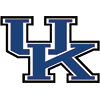Kentucky
30.3% implied probability

+280
2.8 to 1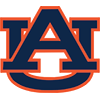Auburn
21.9% implied probability

+500
5 to 1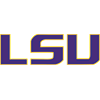Louisiana State
13.9% implied probability

+700
7 to 1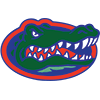Florida
10.4% implied probability

+1400
14 to 1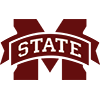Mississippi State
5.5% implied probability

+2000
20 to 1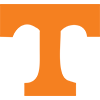Tennessee
4.0% implied probability

+2500
25 to 1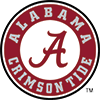Alabama
3.2% implied probability

+2500
25 to 1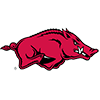Arkansas
3.2% implied probability

+3500
35 to 1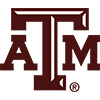Texas A&M
2.3% implied probability

+4000
40 to 1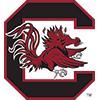South Carolina
2.0% implied probability

+5000
50 to 1Georgia
1.6% implied probability

+10000
100 to 1Missouri
0.8% implied probability

+15000
150 to 1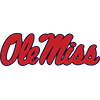Mississippi
0.6% implied probability

+30000
300 to 1Vanderbilt
0.3% implied probability